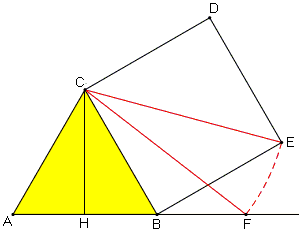# From Equilateral Triangle and Square to Golden Ratio

In a 2011 article, M. Bataille, offered an elegant way of constructing the Golden Ratio via an equlateral triangle and a square.Given an equilateral triangle ABC, erect a square BCDE externally on the side BC. Construct the circle, center C, passing through E, to intersect the line AB at F. Then, B divides AF in the golden ratio.

For a proof, drop a perpendicular CH from C to AB.Assume for convenience that AB = 2, then CH = 3 and CF = CE = 22. By the Pythagorean theorem, in the right triangle CFH,

FH² = CF² - CH² = 8 - 3,

so that FH = 5, making AF = 1 + 5. Thus AF/AB = (1 + 5)/2 = φ, the golden ratio.

### References

1. M. Bataille, Another Simple Construction of the Golden Section, Forum Geometricorum,Volume 11 (2011) 55### Fibonacci Numbers